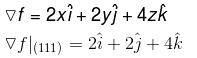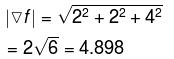Courses

# Vector Calculus NAT Level - 1

## 10 Questions MCQ Test Topic wise Tests for IIT JAM Physics | Vector Calculus NAT Level - 1

Description
This mock test of Vector Calculus NAT Level - 1 for IIT JAM helps you for every IIT JAM entrance exam. This contains 10 Multiple Choice Questions for IIT JAM Vector Calculus NAT Level - 1 (mcq) to study with solutions a complete question bank. The solved questions answers in this Vector Calculus NAT Level - 1 quiz give you a good mix of easy questions and tough questions. IIT JAM students definitely take this Vector Calculus NAT Level - 1 exercise for a better result in the exam. You can find other Vector Calculus NAT Level - 1 extra questions, long questions & short questions for IIT JAM on EduRev as well by searching above.
*Answer can only contain numeric values
QUESTION: 1

###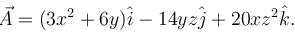Evaluate the line integral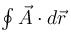from (0, 0, 0) to (1, 1, 1) along the curve x = t, y = t2, z = t3

Solution:

x = t, y = t2, z = t3
dx = dt, dy = 2t dt, dz = 3t2dt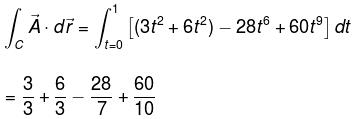= 5

*Answer can only contain numeric values
QUESTION: 2

### Compute the flux of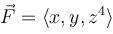across the cone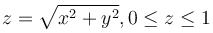(upto three decimal places)

Solution: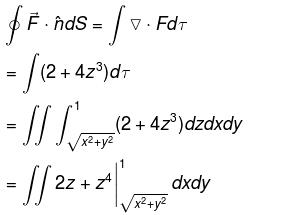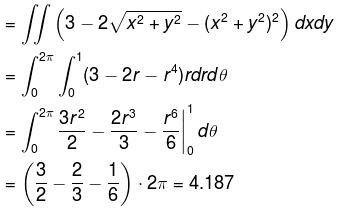*Answer can only contain numeric values
QUESTION: 3

###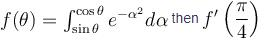Solution: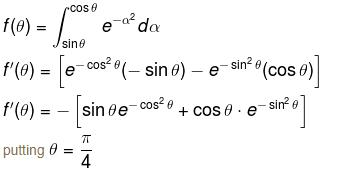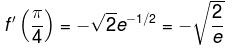= –0.858

*Answer can only contain numeric values
QUESTION: 4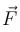is a conservative field and potential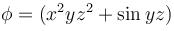any curve C in the path from (0, 0, 1) to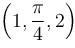evaluate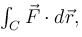upto three decimal places.

Solution:

The conservative field vector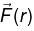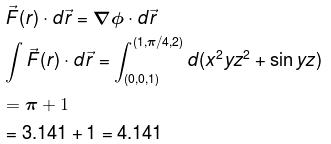*Answer can only contain numeric values
QUESTION: 5

If V = x2y2z the Laplacian of the field V at point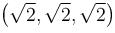is :

Solution: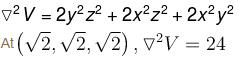*Answer can only contain numeric values
QUESTION: 6

Div grad rm = m(m + 1)rm-α. Find the value of α.

Solution: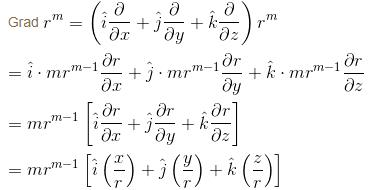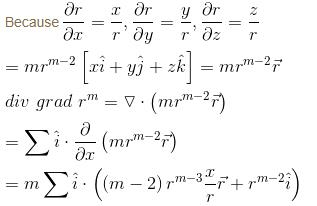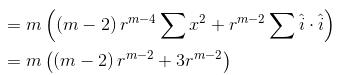= m(m + 1)rm-2
Hence value of  α = 2.

*Answer can only contain numeric values
QUESTION: 7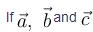are unit vectors satisfying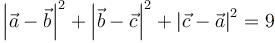then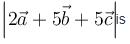Solution: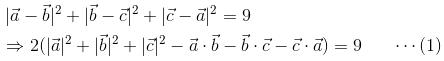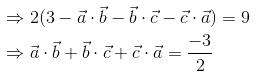⇒ Angle between each pair is 120º

Now,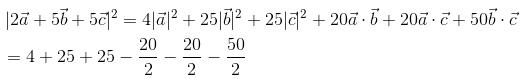= 54 – 10 – 10 – 25 = 9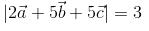*Answer can only contain numeric values
QUESTION: 8

S is the surface of sphere x2 + y2 + z2 = 9, the integral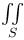[(x + z) dydz + (y + z)dzdx + (x + y)dxdy] is equal to, (upto three decimal places)

Solution: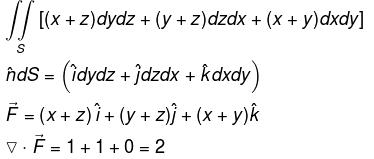By Gauss divergence theorem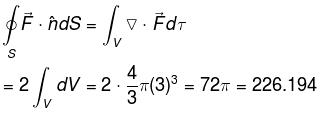*Answer can only contain numeric values
QUESTION: 9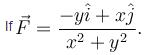Evaluate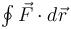around any closed path C. (Ans. upto three decimal places)

Solution: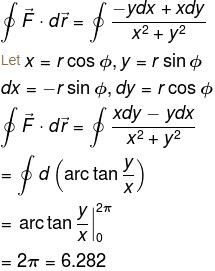*Answer can only contain numeric values
QUESTION: 10

If T(x, y, z) = x2 + y2 + 2z2 defines the temperature at any location (x, y, z) then the magnitude of the temperature gradient point P(1, 1, 1), (upto three decimal places)

Solution: